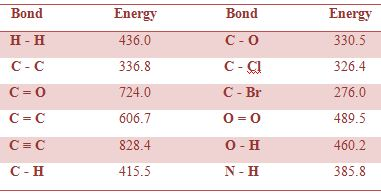Chemistry

# Calculation of Heat of Reaction: Bond EnergyCalculation of Heat of Reaction: Bond Energy

Reactions between covalent compounds actually involve bond breaking and bond forming. The process of breaking bonds between two atoms to form atoms, molecules or free radicals is endothermic. When the same bond is formed from the species obtained in the breaking process, the same amount of energy will be given out according to the law of Lavoisier and Laplace. If the energies for breaking of covalent bonds between different atoms are known, then these values can be used to calculate the enthalpies of reactions. For this purpose a quantity named bond enthalpy is defined as below;

Bond enthalpy, also called bond dissociation energy or bond energy; is defined as the energy necessary to break one mole of bonds between two atoms in a gaseous substance to form gaseous atoms or species.

Bond breaking is an endodermic process while bond forming is an exothermic process.

Bond enthalpy is usually for breaking bonds and is, therefore, positive and expressed in units of kJ mol-1. When the values are stated for 298 K these are referred to as standard bond enthalpy. Every bond between atoms has its own unique strength or bond enthalpy. For example;

H2 (g) → 2H (g);     ∆H0 = 436.0 kJ mol-1

N2 (g) → 2 N (g);    ∆H0 = 945 kJ mol-1

O2 (g) → 2 O (g);    ∆H0 = 489.5 kJ mol-1

An example of a different situation is the breaking four C – H bonds in methane, CH4. One may consider the breaking of the four C – H bonds one at a time. Enthalpy changes per mole for the four steps are shown below;

CH4 (g) → CH3 (g) + H (g);    ∆H0 = + 425 kJ mol-1

CH3 (g) → CH2 (g) + H (g);    ∆H0 = + 470 kJ mol-1

CH (g) → C (g) + H (g);    ∆H0 = + 416 kJ mol-1

CH2 (g) → CH (g) + H (g);    ∆H0 = + 335 kJ mol-1

Again the enthalpy change tot breaking of the first C – H bond in ethane, C2H6, is 410 kJ mol-1. For use of bond enthalpy values in enthalpy calculations the average of the bond dissociation enthalpies under different environments are used.

Bond energies have been calculated from information of the heats of dissociation in the case of elements like hydrogen, oxygen, nitrogen etc. These values are available from a study of the spectra of these compounds. Bond energies may also be calculated from appropriate data obtained from calorimetric and other measurements and use of Hess’s law.

For example let calculate the C-H bond energy. The enthalpy change for the following reaction would he the energy necessary to break four C-H bonds;

CH4 (g) → C (g) + 4 H (g)

The steps below show how the ∆H for this reaction may calculated;

CH4 (g) + 2 O2 (g) → CO2 (g) + 2 H2O (l);     ∆H0 = – 890.3 kJ mol-1

CO2 (g) → C (s) + O2 (g);                               ∆H0 = + 393.5 kJ mol-1

2 H2O (l) → 2 H2 (g) + O2 (g);                        ∆H0 = + 571.8 kJ mol-1

C (s) → C (g);                                                 ∆H0 = + 715.0 kJ mol-1

…………………………………………………………………………………………..

Add, CH4 (g) → C (g) + 4 H (g);                   ∆H0 = 1662 kJ mol-1

Hence C -H bond enthalpy = 1662/4 = 415.5 kJ mol-1.

Bond enthalpy data for some bonds are given in Table;